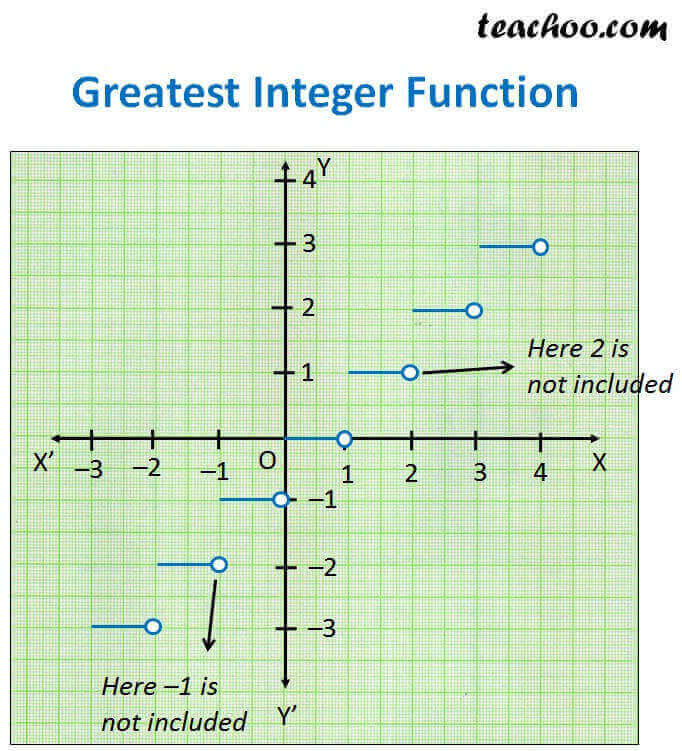Different Functions and their graphs

Chapter 2 Class 11 Relations and Functions
Concept wise

f: R R

f(x) = [x]

[x] is the greatest integer less than or equal to x

 = 0

[0.0001] = 0

[0.1] = 0

[0.9999] = 0

 = 1

[1.01] = 1

[1.2] = 1

[1.99] = 1

[1.9999999] = 1

 = 2

[2.0001] = 2

[2.2] = 2

[2.999] = 2

 = 3

## For negative numbers

#### [–0.1]

Since it is greatest integer less than or equal to x

Integers less than – 0.1 = –1, –2, –3

Greatest Integer less than – 0.1 = –1

∴ [–0.1] = –1

#### [– 0.5]

Since it is greatest integer less than or equal to x

Integers less than – 0.5 = –1, –2, –3

Greatest Integer less than – 0.5 = –1

∴ [–0.5] = –1

#### [–0.999]

Since it is greatest integer less than or equal to x

Integers less than – 0.999 = –1, –2, –3

Greatest Integer less than – 0.999 = –1

∴ [–0.999] = –1

[–1] = –1

#### [–1.1]

Since it is greatest integer less than or equal to x

Integers less than – 1.1 = –2, –3, –4

Greatest Integer less than –1.1 = –2

∴ [–1.1] = –2

#### [–1.999]

Since it is greatest integer less than or equal to x

Integers less than –1.999 = –2, –3, –4

Greatest Integer less than –1.999 = –2

∴ [–1.999] = –2

[–2] = –2

## Now, let us draw the graph

 = 0, [0.0001] = 0, [0.1] = 0, [0.9999] = 0,  = 1

So, for 0 ≤ x < 1, f(x) = 0

 = 1, [1.01] = 1, [1.2] = 1, [1.99] = 1, [1.9999999] = 1,  = 2

So, for 1 ≤ x < 2, f(x) = 1

 = 2, [2.0001] = 2, [2.2] = 2, [2.999] = 2,  = 3

So, for 2 ≤ x < 3, f(x) = 2

[–0.1] = –1, [–0.5] = –1, [–0.999] = –1, [–1] = –1

So, for –1 ≤ x < 0, f(x) = –1

[–1.1] = –1, [–1.999] = –2, [–2] = –2

So, for –2 ≤ x < –1, f(x) = –2

To summarise,

For 0 ≤ x < 1, f(x) = 0

For 1 ≤ x < 2, f(x) = 1

For 2 ≤ x < 3, f(x) = 2

For –1 ≤ x < 0, f(x) = –1

For –2 ≤ x < –1, f(x) = –2Here,

Domain = All values of x = R

Range = All values of y

Since y will have integer values ( … –3, –2, –1, 0, 1, 2, 3, …)

Range = All Integers = Z

Learn in your speed, with individual attention - Teachoo Maths 1-on-1 Class